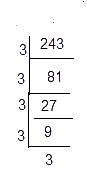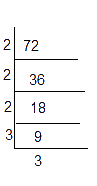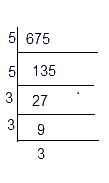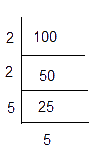# Ex.7.1 Q2 Cubes and Cube Roots - NCERT Maths Class 8

Go back to  'Ex.7.1'

## Question

Find the smallest number by which each of the following numbers must be multiplied to obtain a perfect cube.

(i) $$243$$

(ii) $$256$$

(iii) $$72$$

(iv) $$675$$

(v) $$100$$

Video Solution
Cubes And Cube Roots
Ex 7.1 | Question 2

## Text Solution

What is unknown?

To find the smallest number by which the given number must be multiplied to obtain a perfect cube.

Reasoning:

A number is a perfect cube only when each factor in the prime factorization is grouped in triples.Using this concept, the smallest number can be identified.

Steps:

(i)\begin{align}243 &= \underline {3 \times 3 \times 3} \times 3 \times 3\\243 &= {3^3} \times 3\end{align}

Here, one of the $$3'\rm{s}$$ is  not a triplet.To make it as a triplet, we need to multiply by $$3$$

In that case,

\begin{align}243 \times 3 &= \underline {3 \times 3 \times 3} \times \underline {3 \times 3 \times 3} \\&= {3^3} \times {3^3} \\&= {9^3} = 729\end{align}

is a perfect cube.

Hence, the smallest natural number by which $$243$$ should be multiplied to make a perfect cube is $$3$$.

(ii)

\begin{align}256 &= \underline {2 \times 2 \times 2} \times \underline {2 \times 2 \times 2}\\& \times 2 \times 2\\256 &= {2^3} \times {2^3} \times {2^2}\end{align}

Here one of the $$2’\rm{s}$$ is not a triplet. To make it as a triplet, we need to multiply by $$2.$$

In that case,

\begin{align}256 \times 2 &= \underline {2 \times 2 \times 2} \times \underline {2 \times 2 \times 2} \\&\times \underline {2 \times 2 \times 2} \\&= {2^3} \times {2^3} \times {2^3} \\&= {8^3} = 512\end{align}

is a perfect cube.

Hence, the smallest natural number by which $$256$$ should be multiplied to make a perfect cube is $$2$$.

(iii)\begin{align}72 &= \underline {2 \times 2 \times 2} \times 3 \times 3\\72 &= {2^3} \times {3^2}\end{align}

Here,on of the $$3$$'s is not a triplet. To make it as a triplet, we need to multiply by $$3.$$

In that case,

\begin{align}72 \times 3 &= \underline {2 \times 2 \times 2} \times \underline {3 \times 3 \times 3} \\&= {2^3} \times {3^3} \\&= {6^3} = 216\end{align}

is a perfect cube.

Hence, the smallest natural number by which 72 should be multiplied to make a perfect cube is 3.

(iv)\begin{align}675 &= 5 \times 5 \times \underline {3 \times 3 \times 3} \\675 &= {5^2} \times {3^3}\end{align}

Here, one of the $$5$$'s is not a triplet. To make it as a triplet, we need to multiply by $$5.$$

In that case,

\begin{align}675 &= \underline {5 \times 5 \times 5} \times \underline {3 \times 3 \times 3} \\&= {5^3} \times {3^3} \\&= {15^3} = 3375\end{align}

is a perfect cube.

Hence, the smallest natural number by which $$675$$ should be multiplied to make a perfect cube is $$5$$.

(v)\begin{align}100 &= 2 \times 2 \times 5 \times 5\\100 &= {2^2} \times {5^2}\end{align}

Here both the prime factors are not triplets. To make them triplets we need to multiply by one more  $$2$$ and $$5.$$

In that case,

\begin{align}100 &=\, \underline {2 \times 2 \times 2} \times \underline {5 \times 5 \times 5} \\&= {2^3} \times {5^3} \\&= {10^3} = 1000\end{align}

is a perfect cube.

Hence, the smallest natural number by which 100 should be multiplied to make a perfect cube is $$2 \times 5 = 10.$$

Learn from the best math teachers and top your exams

• Live one on one classroom and doubt clearing
• Practice worksheets in and after class for conceptual clarity
• Personalized curriculum to keep up with school